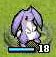# 星之一角

What have you found for these years?

## 2011-05-22

### Haskell/Category theory (0)

(希望沒寫錯的，沒啥時間細琢 ><)

The Joy of Cats

`fmap :: Functor f => (a -> b) -> f a -> f b`

Notice that a functor F : A → B is technically a family of functions;
one from Ob(A) to Ob(B), and for each pair (A,A′) of A-objects, one
from hom(A,A′) to hom(FA,FA′).

map, 然後呢？

`fmap :: Functor f => (a -> b) -> (f a -> f b)`
`a``b` 是 Hask 的 object, 而 `a -> b` 則是 Hask 的 morphism,

Notice that a functor F : A → B is technically a family of functions;
one from Ob(A) to Ob(B), and for each pair (A,A′) of A-objects, one
from hom(A,A′) to hom(FA,FA′).

`f a -> f b` 即是 hom(F Hask, F Hask'). 也就是說，F Hask 和 F Hask'

(
functor law 這邊都先跳過... identity 和 composition (associative?)

)

```map  :: (a -> b) -> (List a -> List b)
List :: Hask -> Lst```
map 是 morphism 的 mapping, List 是 object 的 mapping,

Int, 則 List Int 是一個 Lst 的 object.

A -> B, 這邊的 A 是 Hask (所有 haskell type), 而 B 則是 Lst
(所有 List of something).

category of all categories... (暈)

* * *

 他 concrete example 我只有 set 的東西比較能看懂，

ring, matrix, relation, automata, metric, Banach, 諸如此類...

 這篇比較難，比較簡單的描述可以看 Learn You a Haskell for Great Good!

=> Monad 這樣一路串起來才對。然後 fmap 就是 liftM, 與其把 fmap 看成：
`fmap :: Functor f => (a -> b) -> f a -> f b`

`fmap :: Functor f => (a -> b) -> (f a -> f b)`

「A -> A 的 function 都變成 B -> B」的那一部份。

Applicative 只是單純因為 Monad 比 Applicative 還早加入 haskell.

#### 6 retries:

XOO said...

Lin Jen-Shin (godfat) said...

XOO said...

Lin Jen-Shin (godfat) said...

XOO said...

Functor composition 很多呀。 lists of lists of A 就是一例：List . List : Hask -> Hask。在定義 datatype 的時候也常常用這樣的方式構造新的 type。

>以 haskell 為例，是找出 List a 也是 t (某個 Hask object?) 的 function 之類的？

Lin Jen-Shin (godfat) said...